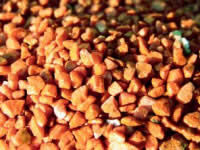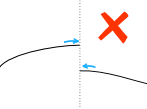# CalculusThe word Calculus comes from Latin meaning "small stone",
Because it is like understanding something by looking at small pieces.

Differential Calculus cuts something into small pieces to find how it changes.

Integral Calculus joins (integrates) the small pieces together to find how much there is.Read Introduction to Calculus or "how fast right now?"

## Limits

Limits are all about approaching. Sometimes you can't work something out directly, but you can see what it should be as you get closer and closer!## Derivatives (Differential Calculus)

The Derivative is the "rate of change" or slope of a function.## Integration (Integral Calculus)

Integration can be used to find areas, volumes, central points and many useful things.## Differential Equations

In our world things change, and describing how they change often ends up as a Differential Equation: an equation with a function and one or more of its derivatives:Second Order Differential Equations:

If you want more Calculus topics covered, let me know which ones.